# New to QlikView

Discussion board where members can get started with QlikView.

Announcements
BARC’s The BI Survey 19 makes it official. BI users love Qlik. GET REPORTNot applicable

## Stacked Bar chart and offset

Dear Community,

I'm trying to created a stacked bar chart using data that captures activity over a day. My raw data has a start time and a duration. I want to stack these in a single column but offset each section so that it starts on the start date and is the size of the duration.I think that I am close but my offset formula is using the "above" expression that I don't 100% understand.

Tags (5)
1 Solution

Accepted Solutions
HighlightedMVP

## Re: Stacked Bar chart and offset

Hi,

another solution could be to create calculated dimensions for date and time parts of the start timestamp and an expression for the bar offsets like

```=RangeSum(Frac(Start),-Above(Frac(Start))-Above(Duration))

```

that calculates the start position of each stacked bar segment.

rangesum() is used because Frac(Start)-Above(Frac(Start))-Above(Duration) would not deliver a value for the first segment of each bar (no above row present). Above() returns the value of the table row before ("above") the current row.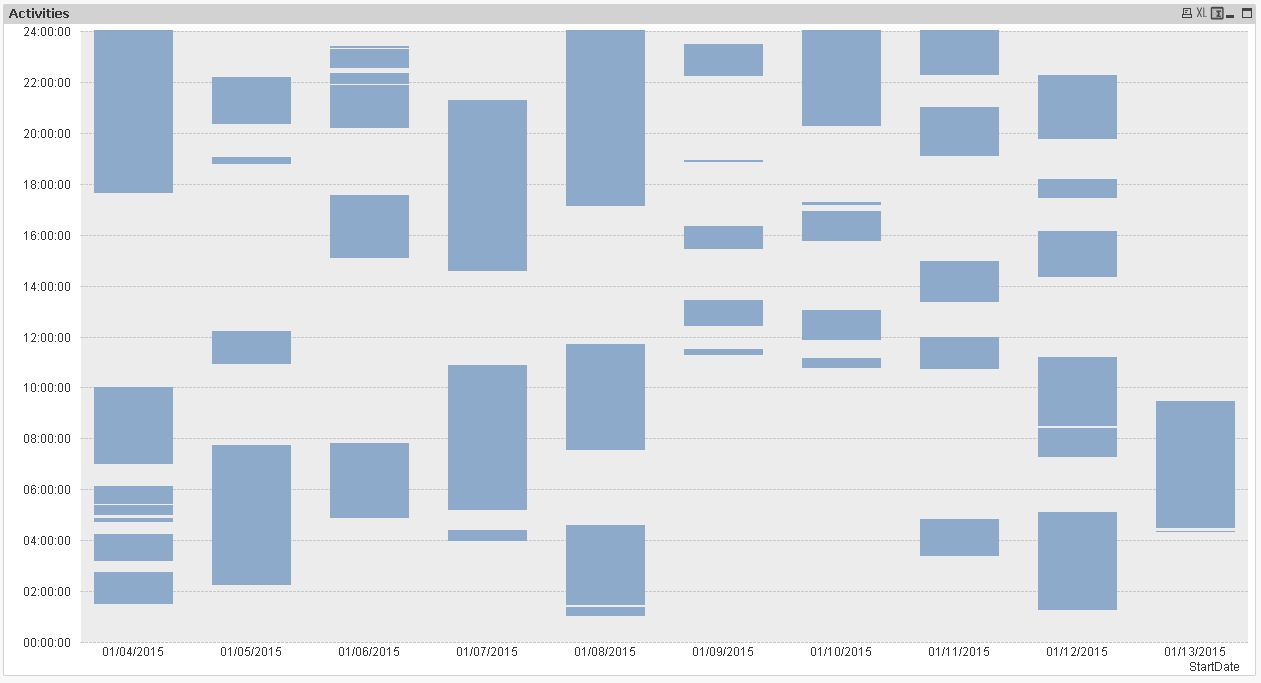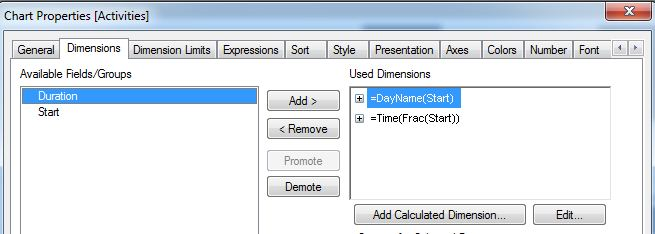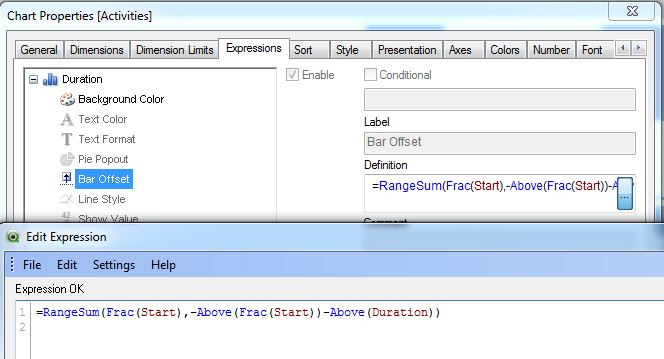hope this helps

regards

Marco

4 Replies
HighlightedMVP

## Re: Stacked Bar chart and offset

Hi,

another solution could be to create calculated dimensions for date and time parts of the start timestamp and an expression for the bar offsets like

```=RangeSum(Frac(Start),-Above(Frac(Start))-Above(Duration))

```

that calculates the start position of each stacked bar segment.

rangesum() is used because Frac(Start)-Above(Frac(Start))-Above(Duration) would not deliver a value for the first segment of each bar (no above row present). Above() returns the value of the table row before ("above") the current row.hope this helps

regards

MarcoNot applicable

## Re: Stacked Bar chart and offset

Hi Marco,

This is so close to exactly what I need. I only have one issue:

1. I want to apply min and max times so that the graph only shows a working day but when I apply these in axis it doesn't appear to work for the minimum?

Also I don't suppose you could provide a brief explanation of how it works so that I might be able to understand things better and trouble shoot if any issues come up.

Many thanks.

Best regards,

DrewMVP

## Re: Stacked Bar chart and offset

Hi,

maybe like this: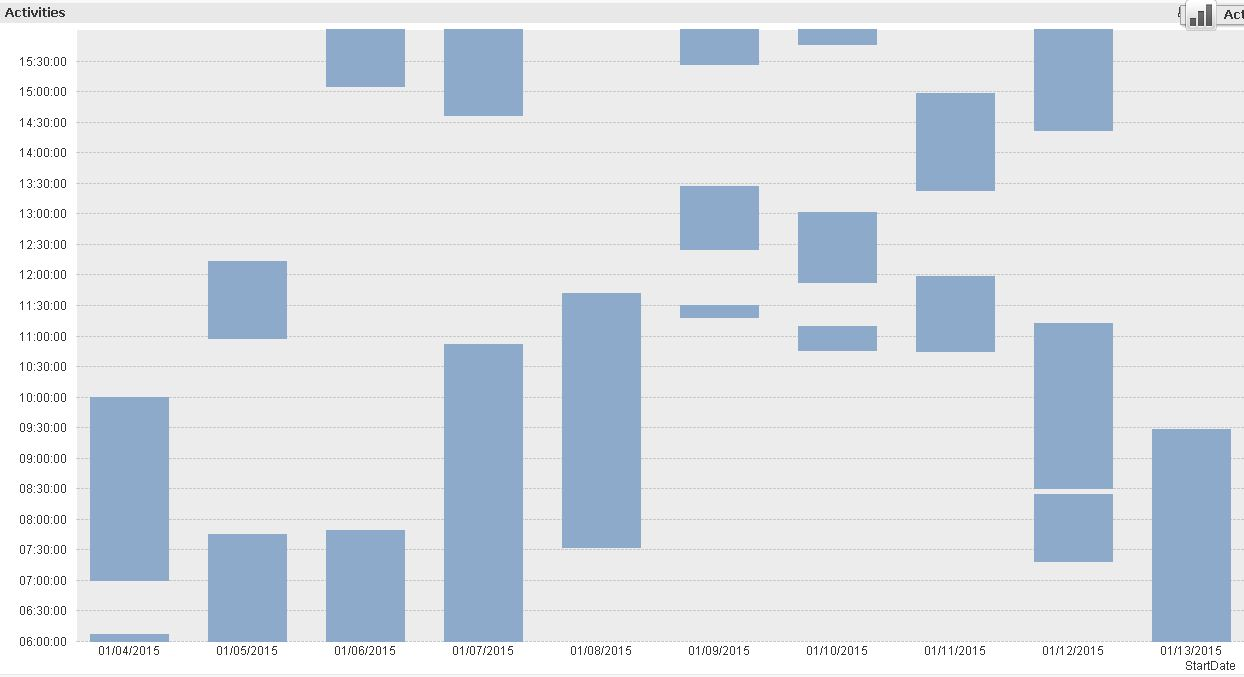did you deselect the "forced 0" check box?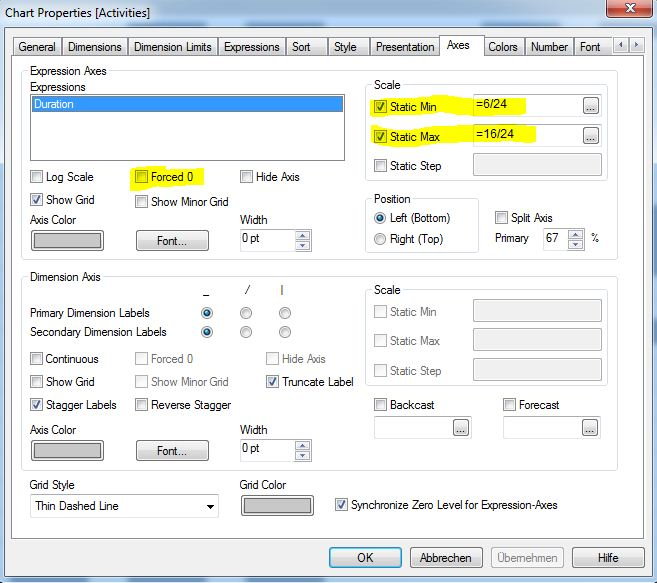As I have only start timestamps and durations in the datamodel, I used

```DayName(Start)
```

as first calculated dimension to extract the date part from the timestamp. DayName rounds of the decimal timestamp value of the start field to full dates and formats the resulting integer as date.

```Time(Frac(Start))
```

as secondary calculated dimension. Frac(Start) extracts the fractional part (containing the time value) of the decimal timestamp value.

Time() formats the resulting number (between 0 and 1) as time.

Since my test data only has one duration for each start timestamp, I just used "Duration" as the only expression.

The bar offset expression (under the dutration expression) defines an offset for each bar as

```=RangeSum(Frac(Start),-Above(Frac(Start))-Above(Duration))
```

which means that one bar starts at a time after the previous bar ends that is defined by "starttime-(starttime+duration of the previous bar)"

hope this makes sense and helps

regards

MarcoNot applicable

## Re: Stacked Bar chart and offset

Hi Marco,

This is brilliant - I am still having a some slight issues with my graph, I think it is because my data is very complex so I am using aggr and mins to get my durations and start times - the graph is plotting quite closely to correct figures but is slightly out. Can you spot anything that is incorrect?

Duration Expression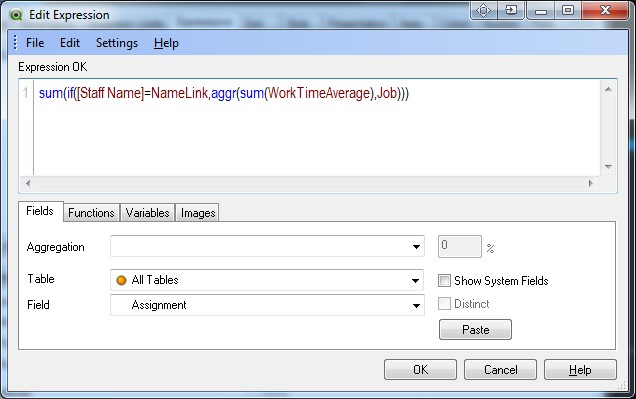Offset Expression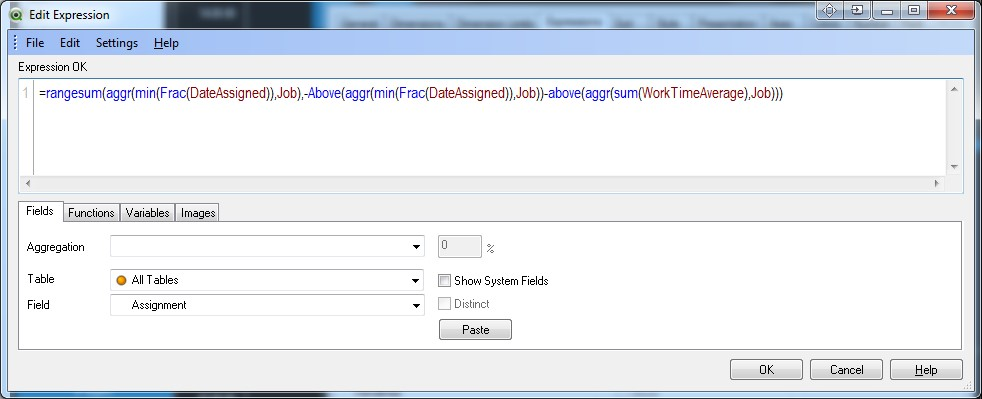The reason for the min and aggr is due to multiple lines for each "Job"

Many thanks for all your help.

Drew